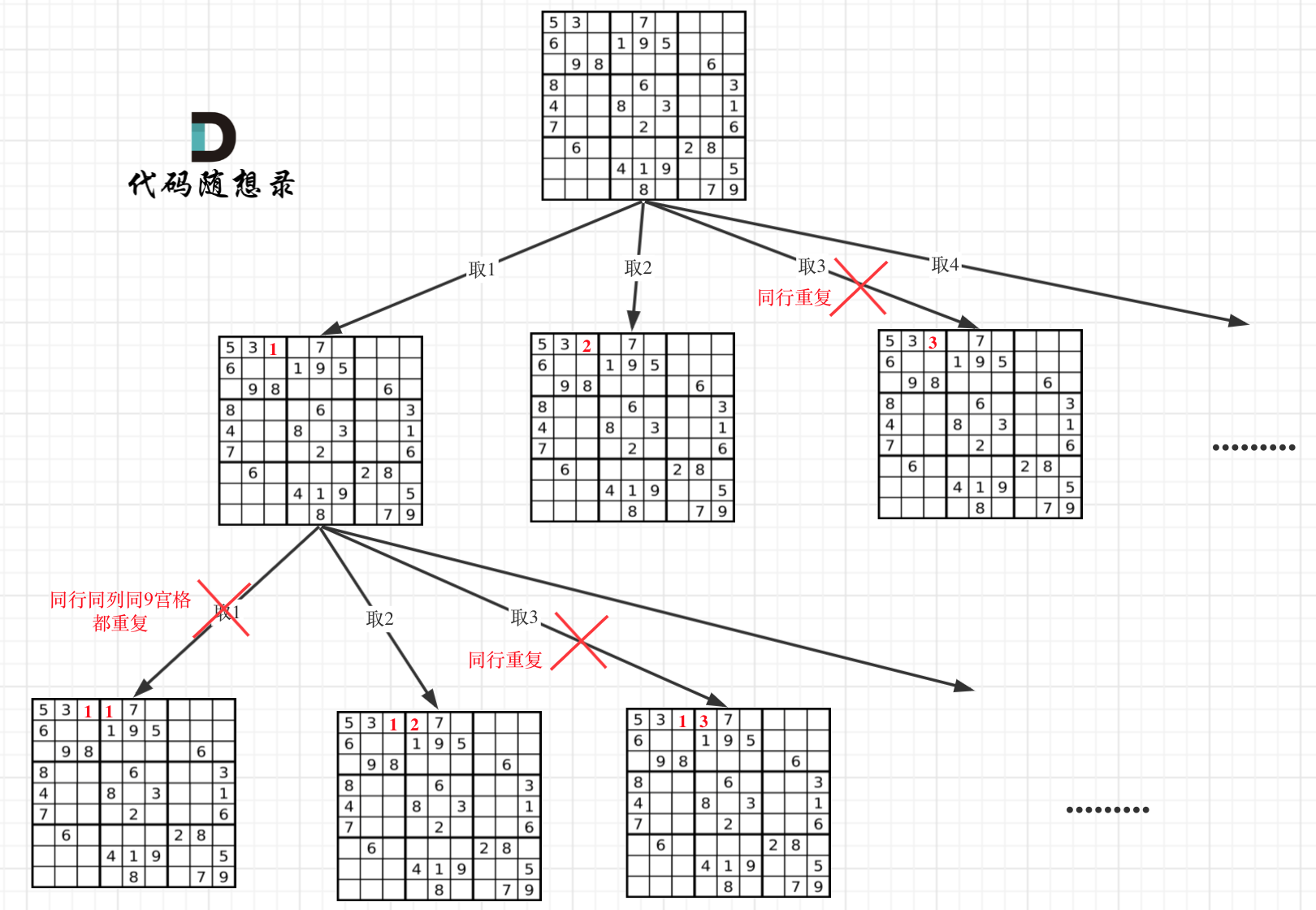# [力扣_37] 解数独

### 题目入口：点击进入

• 深度优先搜索——时间复杂度O(9^9×9),空间复杂度O(9^9*9)[递归栈使用的空间]

３６题是这道题的前篇，如果做过八皇后和N皇后的话，对这道题应该就能有一个大概的思路，我们来看下题目要求：#### DFS

• 在这道题中，坐标会随之而变，给定的二维数组会随之而变，给定的检查数组会随之而变，我们可以整理如下：
• 数独数组、行、列、row检查数组、col检查数组、Samll检查数组。

• 当所有的格子全部迭代完，i=8、j=8，的时候，返回true，代表这个数独已经解完，所有格子都填上了对应的数字

• 什么情况需要回溯呢？当前格子所有数字(1到9)全部测试完，在三个检查数组中全为false的时候，就需要回到上一个状态，同时将上一个状态的三个检查表的状态恢复

• 也就是说，上一个状态填入的数字对应的三个检查表全部恢复成true，而上一个位置必须

回溯成'.'(这个很重要)

• 为什么需要回溯成'.'是因为再所有数字全部填写完并且没有可能的情况下，程序会不断回退，如不回溯 '.' 的话，在下一次再次进入这个格子的时候，就会将它当作已经填入的数字而不进行测试。

• 总之，在写回溯的时候，千万别抱有侥幸心理，觉得下一次的状态会覆盖这次的某一个状态而不进行还原！

• 当当前位置上是【数字】的情况下，我们通过判断边界进行递归，我们需要迭代所有格子，也就是需要遍历整个二维数组，则：

• 当 行 小于 boardSize 当列小于 boardColSize -1 时候，我们将 列坐标不断迭代
• i不变，j+1
• else if 当行小于 7 时，而列==8 时，代表此行的所有列都迭代完毕，就迭代下一行，
• i+1, j 重新为0

• 当当前位置为【空白】的时候，我们循环九种可能【1-9】个数字不断去检查是否满足条件，如果满足，

则将当前位置标记上此数字，然后进行下一次递归，这里注意

• 由于当前位置已经是填入测试的数值，则在进行下一次递归的时候是从当前位置出发到下一个位置，不需要改变坐标。
• 然后判断此递归的返回值是否为ture，如果为true，则返回true到上一层，如果为false则进行回溯

bool dfs(char **board,int boardSize,int boardColSize,int i,int j,bool **row , bool **col, bool ***SamllSudu){
if(i >=boardSize-1 && j >= boardColSize-1 && board[i][j]!='.'){
return true;
}

int SinRow=i/3;
int SinCol=j/3;

if(board[i][j]=='.'){//为空白时
for(int num=1;num<=9;num++){
if(row[i][num]==false || col[j][num]==false || SamllSudu[SinRow][SinCol][num]==false) continue;

row[i][num] = false;
col[j][num] = false;
SamllSudu[SinRow][SinCol][num] = false;
board[i][j]=num+'0';

if(dfs(board,boardSize,boardColSize,i,j,row,col,SamllSudu)){
return true;
}
else{
row[i][num]=true;
col[j][num]=true;
SamllSudu[SinRow][SinCol][num]=true;
board[i][j]='.';
}
}
}
else{//为已填充数字
if (i < boardSize&& j < boardColSize -1) {
return dfs(board, boardSize, boardColSize, i, j + 1, row, col, SamllSudu);
}
else if(i < boardSize-1){
return dfs(board, boardSize, boardColSize, i + 1, 0, row, col, SamllSudu);
}
}
return false;
}

bool dfs(char **board,int boardSize,int boardColSize,int i,int j,bool **row , bool **col, bool ***SamllSudu){
if(i >=boardSize-1 && j >= boardColSize-1 && board[i][j]!='.'){
return true;
}

int SinRow=i/3;
int SinCol=j/3;

if(board[i][j]=='.'){//为空白时
for(int num=1;num<=9;num++){
if(row[i][num]==false || col[j][num]==false || SamllSudu[SinRow][SinCol][num]==false) continue;

row[i][num] = false;
col[j][num] = false;
SamllSudu[SinRow][SinCol][num] = false;
board[i][j]=num+'0';

if(dfs(board,boardSize,boardColSize,i,j,row,col,SamllSudu)){
return true;
}
else{
row[i][num]=true;
col[j][num]=true;
SamllSudu[SinRow][SinCol][num]=true;
board[i][j]='.';
}
}
}
else{//为已填充数字
if (i < boardSize&& j < boardColSize -1) {
return dfs(board, boardSize, boardColSize, i, j + 1, row, col, SamllSudu);
}
else if(i < boardSize-1){
return dfs(board, boardSize, boardColSize, i + 1, 0, row, col, SamllSudu);
}
}
return false;
}

void solveSudoku(char** board, int boardSize, int* boardColSize){
bool **row=(bool**)malloc(sizeof(bool*)*boardSize);
bool **col=(bool**)malloc(sizeof(bool*)*boardSize);

bool ***SamllSudu=(bool***)malloc(sizeof(bool**)*3);

for(int i=0;i < boardSize;i++){
row[i]=(bool*)malloc(sizeof(char)*((*boardColSize)+1));
col[i]=(bool*)malloc(sizeof(char)*((*boardColSize)+1));
for(int k=0;k<=(*boardColSize);k++){
row[i][k]=true;
col[i][k]=true;
}
}

for(int j=0; j < 3; j++){
SamllSudu[j]=(bool**)malloc(sizeof(bool*)*3);
for(int p=0;p<3;p++){
SamllSudu[j][p]=(bool*)malloc(sizeof(bool)*10);
for(int l=0;l<=9;l++){
SamllSudu[j][p][l]=true;
}
}
}

for(int n=0;n<boardSize;n++){
for(int m=0;m<(*boardColSize);m++){
if(board[n][m]!='.'){
int SinRow=n/3;
int SinCol=m/3;
row[n][board[n][m]-'0']=fals
col[m][board[n][m]-'0']=false;
SamllSudu[SinRow][SinCol][board[n][m]-'0']=false;
}
}
}
dfs(board,boardSize,(*boardColSize),0,0,row,col,SamllSudu);
return;
}

## 本文链接：

https://nullcode.fun/84.html
1 + 9 =